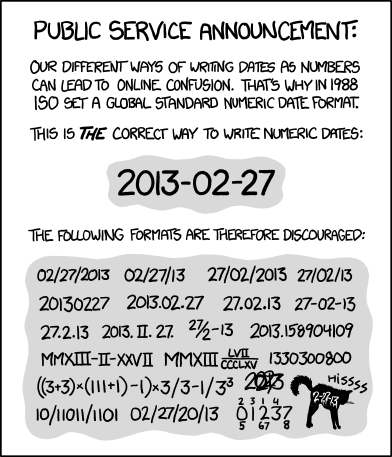# How to extract full number from a string?

Best illustrated with an example:

``````         |       | desired output
12/10/12 | + 12m | 12/10/13
01/04/13 | - 4m  | 09/04/12
07/21/15 | - 3m  | 04/21/15
03/20/18 | + 24m | 03/20/20
``````

I want to extract the number each cell of column 2 and add/subtract that month
to the date in column 1 depending on the first "“word” (delimited by a space) of
the string (+/-).

The second word is always the month indicator and ends with an “m”.

Obviously it would better to simply use e.g. negative/positive numbers and
imply the number of months to add/subtract, but for the purposes of this
specific example, I want to be able to do this very basic string parsing and
work from there.

I am so far able to extract the first character using: e.g. `MID(B2,1,1)="+"`.

Much appreciated.

`=VALUE(REGEX(B2;"([+-])\s*(\d+).*";"\$1\$2"))`

The end result in `C2` would be

`=EDATE(A2;VALUE(REGEX(B2;"([+-])\s*(\d+).*";"\$1\$2")))`